# Smart Communicating Irrigation System

Automatic and controled plant irrigation system with data monitoring through internet, locally or via SMS + alerts.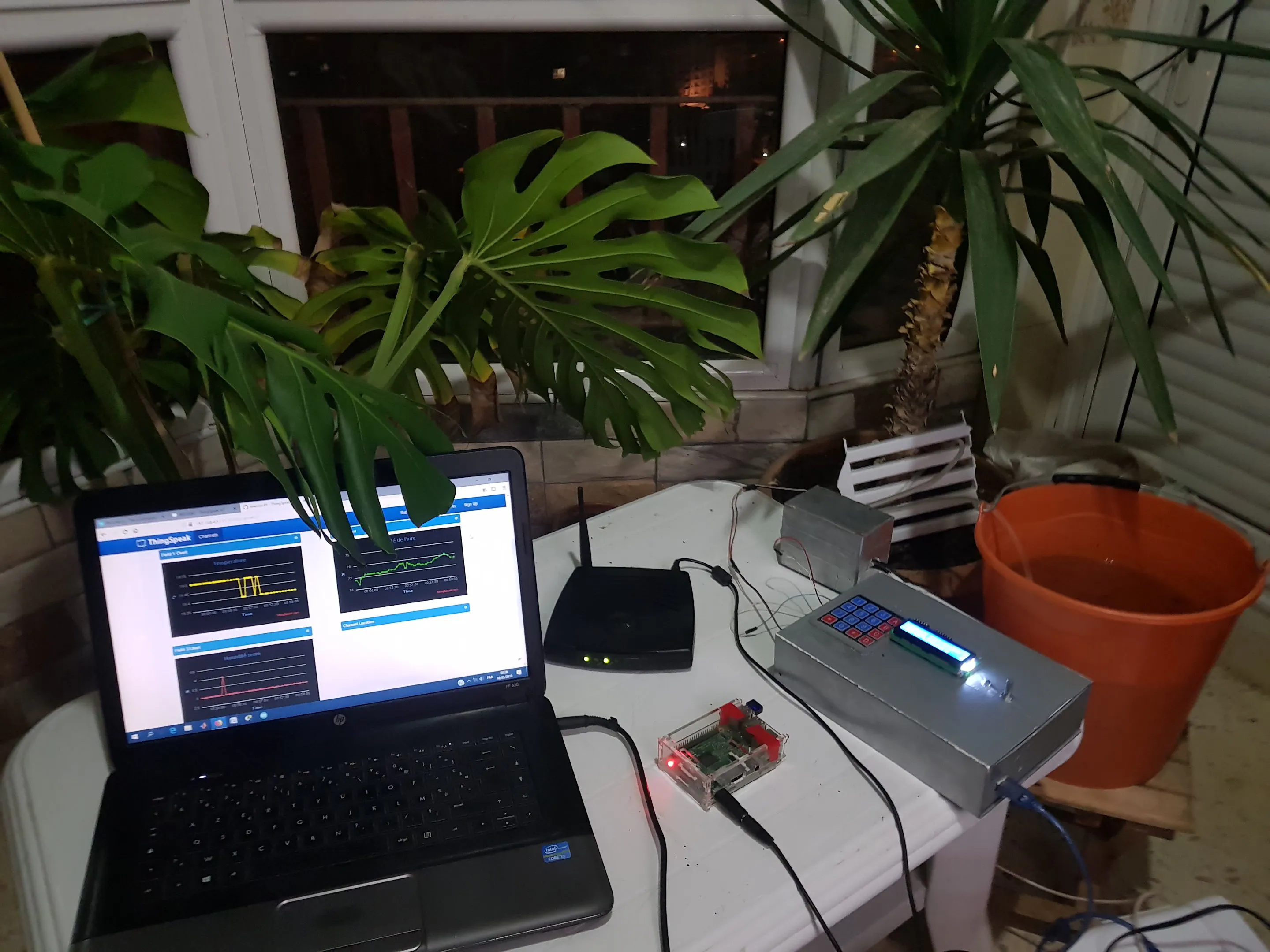## Things used in this project

### Hardware components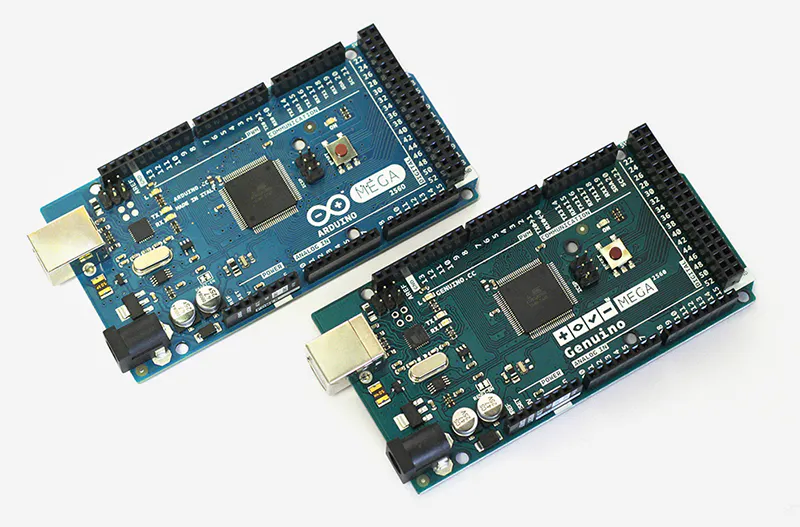Arduino Mega 2560 & Genuino Mega 2560
×1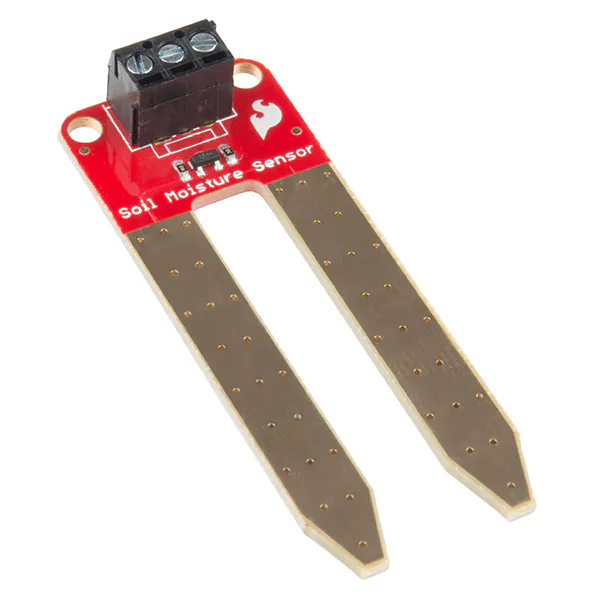SparkFun Soil Moisture Sensor (with Screw Terminals)
×1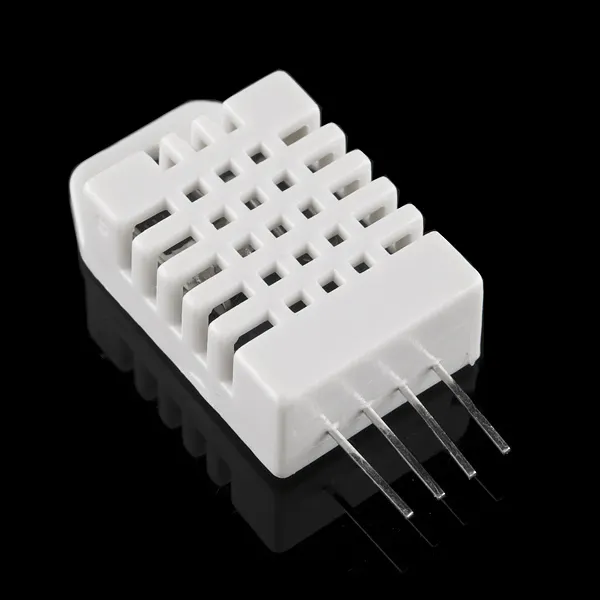DHT22 Temperature Sensor
×1Arduino Mega 2560 & Genuino Mega 2560
×1
 GSM sim800l
×1
 MicroSD Module (Generic)
×1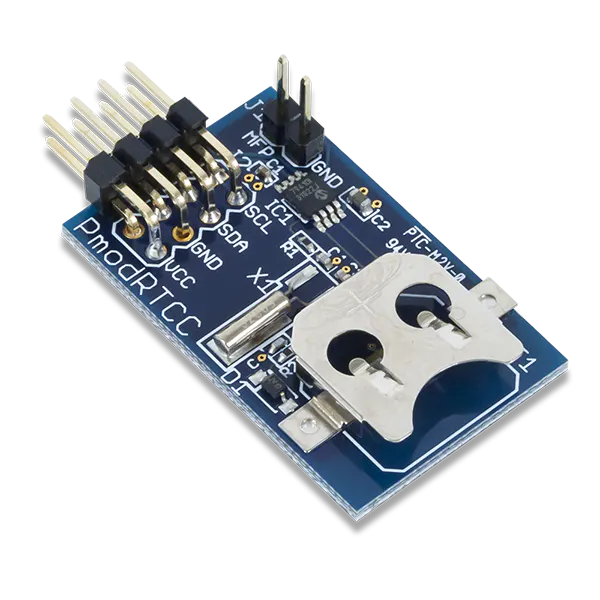Digilent Pmod RTCC
×1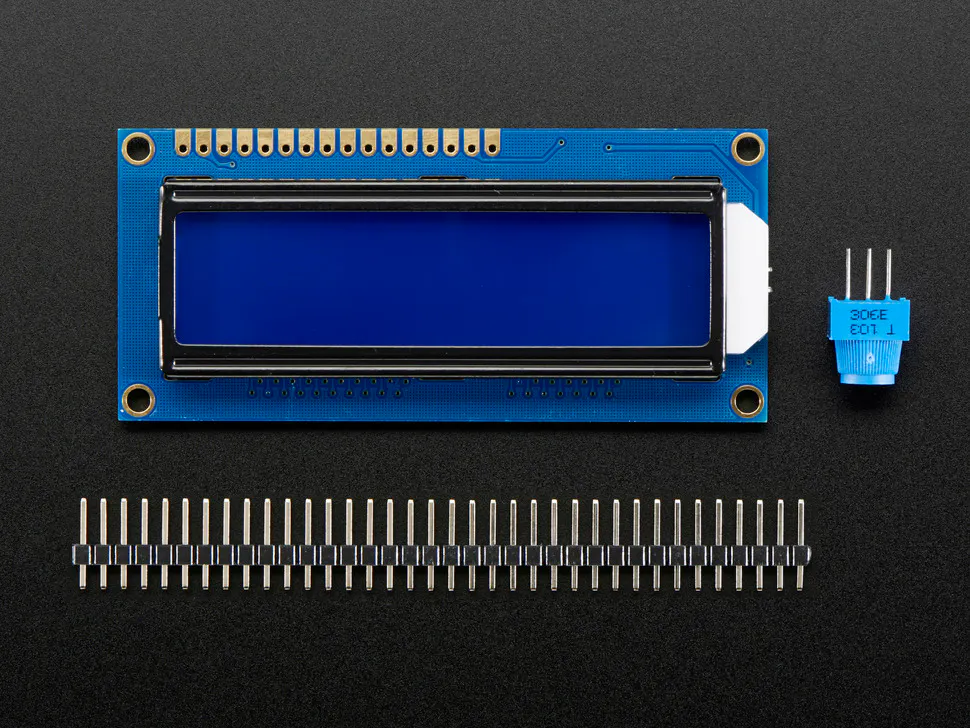Adafruit Standard LCD - 16x2 White on Blue
×1
×1Raspberry Pi 3 Model B
×1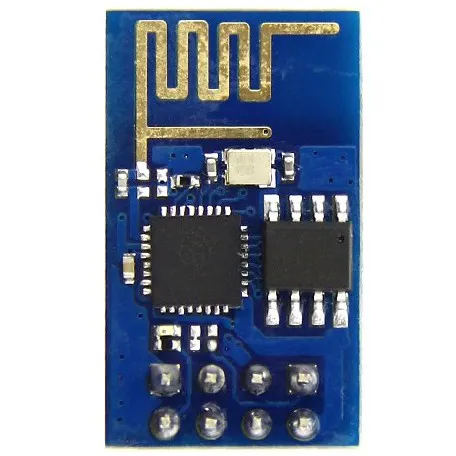Espressif ESP8266 ESP-01
×1

### Software apps and online servicesArduino IDE

## Code

### project's code

Arduino
follow the pins as in the code or modify it as your modules pins
```#include "DHT.h"
#define DHTPIN 32                       // Digital Pin 32
#define DHTTYPE DHT22    // We are Using DHT22
#include <string.h>
#include <SoftwareSerial.h>
#include <Sim800l.h>
#include <SD.h>//SD Card Library
Sim800l Sim800l;  //to declare the library
#include <DS3231.h>
DS3231  rtc(SDA, SCL);

//String Host_Name = "ANON0NOSSe";         // Edit Host_Name
String apiKey = "5Z1WCK3CVIASN5TW";    // Edit this API key according to your Account
String apiKey1 = "WQNEJ9CE6V2U25EX"; // API LOCAL-------------------
String Host_Name = "USTHB";         // Edit Host_Name

const int pompe=8;
const byte inter=38;
String temp;
String MyString;
String mystring;

int c=0;
int n =0;
int i=0;
int p=0;
int m=0;
//String text;     // to storage the text of the sms
uint8_t index;   // to indicate the message to read.
char* text;
char* number;
int posision = 0;
int ledV =40;
int ledR = 42;
int ledB = 46;
int CS_pin = 53;
SoftwareSerial ser(12, 9);// RX, TX
DHT dht(DHTPIN, DHTTYPE);              // Initialising Pin and Type of DHT

#include <LiquidCrystal.h>
LiquidCrystal lcd(47, 45, 43, 41, 39, 37);

char keys = {
{'1','2','3','A'},
{'4','5','6','B'},
{'7','8','9','C'},
{'*','0','#','D'}
};

byte rowPins = {29,28,27,26};  //connect to the row pinouts of the keypad
byte colPins = {25,24,23,22}; //connect to the column pinouts of the keypad

int pinpower= 48;
int pinpower1=49;
int pinpower2=6;
int GND=7;
int gnD=4;
int gnd= 36 ;
int GnD=35;
int VCC=44;

long id = 1;

void setup() {

///////////////////////////////ALIMENTATION//////////////////////////////
pinMode(VCC,OUTPUT);
digitalWrite(VCC,1);
pinMode(GnD,OUTPUT);
digitalWrite(GnD,0);
pinMode(pinpower,OUTPUT);
digitalWrite(pinpower,1);
pinMode(pinpower1,OUTPUT);
digitalWrite(pinpower1,1);
pinMode(pinpower2,OUTPUT);
digitalWrite(pinpower2,1);
pinMode(gnd,OUTPUT);
digitalWrite(gnd,0);
pinMode(GND,OUTPUT);
digitalWrite(GND,0);
pinMode(gnD,OUTPUT);
digitalWrite(gnD,0);
pinMode(inter,OUTPUT);
digitalWrite(inter,1);

////////////////////////////////////////////////////////////////////////

lcd.begin(16, 2);

Serial.begin(9600);

pinMode(CS_pin, OUTPUT);

// Serial.begin(9600); // only for debug the results .
if (!SD.begin(CS_pin))
{
Serial.println("Card Failure");

return;
}

File logFile = SD.open("LOG.csv", FILE_WRITE);
if (logFile)
{
logFile.println(", , , ,"); //Just a leading blank line, incase there was previous data
String header = "ID, humidity, Temp, time, humid terre, day, date ";
logFile.close();
}
else
{
Serial.println("Couldn't open log file");
}

ser.begin(115200);
//ser.println("AT+RST");
ser.println("AT+CIOBAUD=9600");
delay(4000);
// enable software serial
ser.begin(9600);
pinMode(ledV, OUTPUT);
pinMode(ledB, OUTPUT);
pinMode(ledR, OUTPUT);

dht.begin();

rtc.begin();
/////////////////////////////////REGLAGE RTC //////////////////////////////////////
//rtc.setDOW(SUNDAY);     // Set Day-of-Week to SUNDAY                          //
//rtc.setTime(14, 20, 00);     // Set the time to 12:00:00 (24hr format)        //
// rtc.setDate(29, 3, 2018);   // Set the date to January 1st, 2014            //
////////////////////////////////////////////////////////////////////////////////

char inv ='"';
String cmd1 = "AT+CWJAP";
cmd1+= "=";
cmd1+= inv;
cmd1+= Host_Name;
cmd1+= inv;
cmd1+= ",";
cmd1+= inv;
cmd1+= inv;
ser.println(cmd1);                // Connecting ESP8266 to your WiFi Router

pinMode(ledV,OUTPUT);
pinMode(ledB,OUTPUT);
pinMode(ledR,OUTPUT);

}

void loop() {

attachInterrupt(digitalPinToInterrupt(3), interrupt,HIGH);
//////////////////////////////////////////////////////////////////Security/////////////////////////////////////////////////////////////////////////////////////////////////////////////////////
while (n!=1)
{

//lcd.clear();
lcd.setCursor(0, 0);
lcd.print("   Welcome");
lcd.setCursor(0, 1);
delay(100);

if(key == '*' || key == '#' || key == 'A' ||       //define invalid keys
key == 'B' || key == 'C' || key == 'D'){

posision=0;

lcd.clear();
lcd.setCursor(0, 0);
lcd.print("  Invalid Key!");
digitalWrite(ledV, 0);
digitalWrite(ledR, 1);
digitalWrite(ledB, 0);
delay(1000);

digitalWrite(ledV, 0);
digitalWrite(ledR, 0);
digitalWrite(ledB, 0);

lcd.clear();

}

posision ++;
}

if(posision == 4){

lcd.clear();
lcd.setCursor(0, 0);
lcd.print("*** Verified ***");
n=1;
digitalWrite(ledV, 1);
digitalWrite(ledR, 0);

digitalWrite(ledB, 0);
delay(2000);
lcd.clear();
lcd.setCursor(0,0);

lcd.print("press 0 for help");
delay(50);
}

}

/////////////////////////////////////////////////////////////////////////////////////////////////////////////////////////////////////////////////////////////////////////////////////////////////

if(n==1)
{
//Serial.println(sensorValue);
sensorValue= map(sensorValue,200,1024,100,0);
delay(200);
String dataString = String(id) + ", " + String(h) + ", " + String(t)+ ", " + String(sensorValue)+", " + String (rtc.getTimeStr())+ ", " + String (rtc.getDOWStr())+ ", " + String (rtc.getDateStr());

//Open a file to write to
//Only one file can be open at a time
File logFile = SD.open("LOG.csv", FILE_WRITE);
if (logFile)
{
logFile.println(dataString);
logFile.close();
Serial.println(dataString);
}
else
{
Serial.println("Couldn't open log file");
}
// Wait one second before repeating :)
delay (50);
//Increment ID number
id++;

digitalWrite(ledV, 0);
digitalWrite(ledR, 0);
digitalWrite(ledB, 0);

/////////////////////////////////////////
rtc.getDOWStr();
// Send date
rtc.getDateStr();
// Send time
rtc.getTimeStr();

//int index=1; // first position in the prefered memory storage.
//String text;
//lcd.print(text);
//delay(5000);
//lcd.clear();

////Parti____lcd///////

if (key=='0'){
i=8;
lcd.clear();
}
if (i==8)
{

digitalWrite(ledV, 1);
digitalWrite(ledR, 0);

digitalWrite(ledB, 0);

lcd.setCursor(2, 0);
lcd.print("1-humidite");
lcd.setCursor(2, 1);
lcd.print("2-temperature");

lcd.setCursor(16, 0);
lcd.print("3-humid terre");
lcd.setCursor(16, 1);
lcd.print("D-date");

lcd.setCursor(30, 0);
lcd.print("#-send sms ");
lcd.setCursor(29, 1);
lcd.print("*-send wifi ");
lcd.scrollDisplayLeft();

delay(400);

}
digitalWrite(ledV, 0);
digitalWrite(ledR, 0);

digitalWrite(ledB, 0);

if (key=='1'){
i=1;
lcd.clear();
}
if(i==1){

lcd.setCursor(0,0);
lcd.print("Humidity:");
lcd.print(h);
lcd.print("%");
lcd.setCursor(0,1);
lcd.print(rtc.getTimeStr());

}

if (key =='2'){
i=2;
lcd.clear(); }
if(i==2){

lcd.setCursor(0,0);
lcd.print("temp :");
lcd.print(t);
lcd.setCursor(0,1);
lcd.print(rtc.getTimeStr());
}

if (key =='C'){
i=10;
lcd.clear();
}
if(i==10){
lcd.setCursor(0,0);
lcd.print (dataString);
lcd.scrollDisplayLeft();
delay(400);
}

if (key=='3'){
i=3;
lcd.clear();
}
if(i==3){
lcd.setCursor(0,0);
lcd.print("humid terre:");
lcd.print(sensorValue);
lcd.print("%");
lcd.setCursor(0,1);
lcd.print(rtc.getTimeStr());
}

if(key=='D')
{
i=6;
lcd.clear();
}
if(i==6){
lcd.setCursor(33,0);
lcd.print( "Date:");
lcd.setCursor(4,0);
lcd.print( rtc.getDOWStr());

lcd.setCursor(2,1);
lcd.print(rtc.getDateStr())  ;
lcd.setCursor(18,0);
lcd.print( "TIME ");

lcd.setCursor(20,1);
lcd.print(rtc.getTimeStr());
lcd.print(" ");

lcd.scrollDisplayLeft();
delay(500);

}

if (key=='#'){
i=7;
}
if (i==7)
{

lcd.clear();
lcd.print("sending SMS");
mystring = String (h,0);
MyString= String (t,0);
temp= (rtc.getTimeStr());
MyString = ("temperature: "+MyString +"*C "+ "humidity: "+ mystring+" %"+"  ---  " "time :"+ temp);

int str_len = MyString.length() + 1;
char char_array[str_len];

MyString.toCharArray(char_array,str_len);

Sim800l.begin(); // initializate the library.
number="0658564816"; //change to a valid number.
//text=TEXT;
//

Sim800l.sendSms(number,char_array);
delay(3000);

}

if(key=='B')
{p=1;
lcd.clear();
lcd.print("mode manuel");
pinMode(pompe,OUTPUT);
digitalWrite(pompe,0);
delay(1000);
}
if(key=='A'){
p=2;
lcd.clear();
lcd.print("mode auto");
delay(1000);
}
if(p==1)
{
i=5;
key='5';
}

if(p==2){
if(sensorValue<40)
{
digitalWrite(ledV, 1);
digitalWrite(ledR, 0);
digitalWrite(ledB, 0);

pinMode(pompe,OUTPUT);
digitalWrite(pompe,1);
lcd.clear();
lcd.setCursor(5,0);
lcd.print("Arrosage");
lcd.setCursor(5,1);
lcd.print("en cours");

digitalWrite(ledV, 0);
digitalWrite(ledR, 0);
digitalWrite(ledB, 0);
}
else
{
pinMode(pompe,OUTPUT);
digitalWrite(pompe,0);
}
}
if (key=='5')
{
lcd.clear();
lcd.print("mode internet");
m=1;
}
//////////////////////////
//////////
if (key=='*')

{

i=5;
lcd.clear();
lcd.setCursor(0,0);
lcd.print("wifi send");
lcd.setCursor(0,1);
lcd.print ("temp and humid");
delay(3000);
}
if(i==5){

digitalWrite(ledV, 0);
digitalWrite(ledR, 0);
digitalWrite(ledB, 1);

lcd.clear();
lcd.setCursor(0,0);
lcd.print("Humidite: ");
lcd.print(h);
lcd.print("%");
lcd.setCursor(0,1);
lcd.print("Temperature:");
lcd.print(t);
lcd.print("*C");

ser.begin(115200);
//ser.println("AT+RST");
ser.println("AT+CIOBAUD=9600");
delay(20);
// enable software serial
ser.begin(9600);

String state1=String(t);                 // Converting them to string
String state2=String(h);              // as to send it through URL
String state3=String(sensorValue);
String cmd = "AT+CIPSTART=\"TCP\",\"";          // Establishing TCP connection
if(m==0){
c=0;
}
if (m==1,c==1){
//  ------------------------------------------------------------------------------------------------
cmd += "184.106.153.149";   // api.thingspeak.com
cmd += "\",80";                                 // port 80

}

else{
//---------------------------------------------------------------------------------------------
cmd += "192.168.43.111"; // api.THINGSPEAKSTANDALONE--------------
cmd += "\",3000";  //--------------port local
// ------------------------------------------------------------------------------------------------

}

ser.println(cmd);

// AT+CIPSTART="TCP",184.106.153.149,"80"

//enleve par  moi
if(ser.find("Error")){
ser.println("AT+CIPSTART error");
return;
}

String getStr = "GET /update?api_key=";    // prepare GET string
if (m==1,c==1){
getStr += apiKey;
c--;
}
else {
getStr += apiKey1;
c++;
}

getStr +="&field1=";
getStr += String(state1);                       // Temperature Data
getStr +="&field2=";
getStr += String(state2);                       // Humidity Data
//getStr += "\r\n\r\n";
////////////essai//////////
getStr +="&field3=";
getStr += String(state3);                       // Humidity terre Data
getStr += "\r\n\r\n";
//////////////
cmd = "AT+CIPSEND=";
cmd += String(getStr.length());                // Total Length of data
ser.println(cmd);

if (ser.find(">"))
{
ser.println(getStr);
}
else{
ser.println("AT+CIPCLOSE");                  // closing connection
delay(20);
}
if(p==1)
{
talkback();
}
digitalWrite(ledV, 0);
digitalWrite(ledR, 0);
digitalWrite(ledB, 0);

}

//String dataString = String(id) + ", " + String(h) + ", " + String(t)+ ", " + String (rtc.getTimeStr());

//////////////////////////////////////

if(h>=95)
{
lcd.clear();
digitalWrite(ledV, 0);
digitalWrite(ledR, 1);
digitalWrite(ledB, 0);

Sim800l.begin(); // initializate the library.
text="ALERTE HUMIDITE";  //text for the message.
number="0658564..."; //change to a valid number.
Sim800l.sendSms(number,text);
lcd.clear();
lcd.print(text);
delay(5000);
}

}

}

void interrupt()
{
i=1;

}

void talkback()
{
//////////////////////// START: POMPE_ON TALKBAK ////////////////////////////
ser.println("AT+CIPMUX=0\r\n");    // Set MUX=0
delay(200);

// TCP connection
String cmd = "AT+CIPSTART=\"TCP\",\"";   // TCP connection with https://thingspeak.com server
cmd += "184.106.153.149";                // api.thingspeak.com
cmd += "\",80\r\n\r\n";                  // Port No. = 80

ser.println(cmd);                    // Send above command to Rx1, Tx1

delay(200);

if(ser.find("ERROR"))                // If returns error in TCP Connection
{
Serial.println("AT+CIPSTART error");   // Display error msg to PC
return;
}

// prepare GET string
// Replace <TalkBack_ID>, <Command_ID> and <API_Key> by actual values here
String getStr = "GET /talkbacks/24264/commands/12511276?api_key=CX8DMME15ZE4QP3R\r\n\r\n";  //POMPE_ON Get a TalkBack Command

// send data length
cmd = "AT+CIPSEND=";
cmd += String(getStr.length());
cmd+="\r\n";

ser.println(cmd);                   // Send Data length command to Tx, Rx

delay(100);                             // wait for 0.1 Sec

if(ser.find(">"))                   // If prompt opens //verify connection with cloud
{
Serial.println("Request Sent");       // Display confirmation msg to PC
ser.print(getStr);                // Send GET String to Rx, Tx
}
else
{
ser.println("AT+CIPCLOSE");       // Send Close Connection command to Rx1, Tx1
Serial.println("Closing Connection"); // Display Connection closed command on PC
}
delay(300);

if(ser.available()>0)               // Check Buffer
{
delay(200);                          // wait for 1 Sec
while(ser.available())            // Till Buffer is not Empty
{
}
Serial.println("____________");       // Display msg to PC
Serial.println("Response: ");         // Display msg to PC

Serial.print(recd);    // Display Received msg to PC

}

if(find_text("POMPE_ON",recd)==1)         // Check if POMPE_ON msg received
digitalWrite(pompe,HIGH);               // POMPE ON
if(find_text("POMPE_ON",recd)==1) {
lcd.clear();
lcd.print("pompe on");}

//////////////////////// END: POMPE_ON TALKBAK ////////////////////////////

//////////////////////// START: POMPE_OFF TALKBACK ////////////////////////////
ser.println("AT+CIPMUX=0\r\n");    // Set MUX=0
delay(200);

// TCP connection
cmd = "AT+CIPSTART=\"TCP\",\"";          // TCP connection with https://thingspeak.com server
cmd += "184.106.153.149";                // api.thingspeak.com
cmd += "\",80\r\n\r\n";                  // Port No. = 80

ser.println(cmd);                    // Send above command to Rx, Tx

delay(200);

if(ser.find("ERROR"))                // If returns error in TCP Connection
{
Serial.println("AT+CIPSTART error");   // Display error msg to PC
return;
}

// prepare GET string
// Replace <TalkBack_ID>, <Command_ID> and <API_Key> by actual values here
getStr = "GET /talkbacks/24264/commands/12430486?api_key=CX8DMME15ZE4QP3R\r\n\r\n";   //POMPE_OFF Get a TalkBack Command

// send data length
cmd = "AT+CIPSEND=";
cmd += String(getStr.length());
cmd+="\r\n";

ser.println(cmd);                   // Send Data length command to Tx1, Rx1

delay(100);                             // wait for 0.1 Sec

if(ser.find(">"))                   // If prompt opens //verify connection with cloud
{
Serial.println("Request Sent");       // Display confirmation msg to PC
ser.print(getStr);                // Send GET String to Rx1, Tx1
}
else
{
ser.println("AT+CIPCLOSE");       // Send Close Connection command to Rx1, Tx1
Serial.println("Closing Connection"); // Display Connection closed command on PC
}
delay(300);

if(ser.available()>0)               // Check Buffer
{
delay(200);                          // wait for 1 Sec
while(ser.available())            // Till Buffer is not Empty
{
}
Serial.println("____________");       // Display msg to PC
Serial.println("Response: ");         // Display msg to PC

Serial.print(recd);                   // Display Received msg to PC

}

if(find_text("POMPE_OFF",recd)==1)        // Check if POMPE_OFF msg received
digitalWrite(pompe,LOW);                // POMPE OFF
if(find_text("POMPE_OFF",recd)==1){
lcd.clear();
lcd.print("pompe off");}
//////////////////////// END: POMPE_OFF TALKBAK ////////////////////////////

delay(200);
}

int find_text(String sr, String recd)      //function to serach a Substring in Received msg
{
int foundpos = -1;
for (int i = 0; (i < recd.length() - sr.length()); i++)
{
if (recd.substring(i,sr.length()+i) == sr)
{
foundpos = 1;
}
}
return foundpos;
}

```